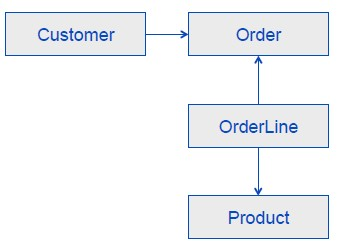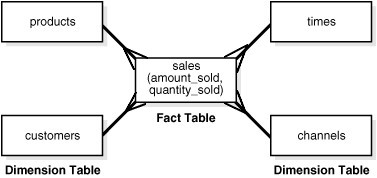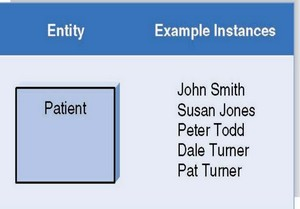# Dimensional Data Modeling - Degenerate Dimension of Fact dimension (i.e. event/header entity)

## Fact Dimension

In a dimensional model, you may find a dimension table:

• with a cardinality (distinct value is higher than 10,000). A high cardinality means generally that the values are different for almost every transaction. For example, order ID, order line ID, and notes).
• which has a many-to-one relationship with the fact table close to a one-to-one and then grows roughly at the same rate as the fact table

This kind of tables are often called:

• fact dimensions
• event facts
• factless fact table

as they contain often attributes that are related to the event of the fact table without containing a measure.

In an OLTP environment, you may find them as header tables of transaction lines such as sales, and invoices.

In this dimension table, you may find only two different data type attributes:

• a character data type known/referenced as textual facts. Textual facts are useful for grouping together related fact table rows, such as all the rows that are related to a particular invoice number.
• a time data type
• but you may not find a number attribute that you can aggregate (i.e. with a SUM for instance). if it's already an aggregation of another table (such as a sold for a customer).

## Degenerate Dimension

To avoid an expensive join between this fact dimension and the fact table, the attribute of this dimension are degenerate i.e. moved from the dimension to the fact table and can then be classified as:

• Fact attributes
• Degenerate attribute

For example of degenerate attribute:

• Order Numbers,
• Invoice Number,
• Credit-Debit Indicator
• etc

Do not confuse! Degenerate dimensions can in articles referred to a fact dimension.

## Pros and Cons of the degeneration

### Pros

• Avoid a expensive join between two tables with a one-to-many relationship but which is close of a one-to-one relationship
• By degenerating a date dimension attribute, you avoid the need for a join between the event dimension and a time dimension (which enable the drill through the time hierarchy)

### Cons: Increase the size of the fact table

By moving the dimensional attribute in the fact table, you increase the need for storage and you may use a partial_degeneration

## Partial degeneration

To overcome the increase of capacity needs when you degenerate a complete fact dimension, you can make a partial degeneration of the fact dimension by choosing to degenerate only a set of attribute with a high analytic value (i.e often used in reporting).

While it is generally not considered good dimensional modeling practice to create a dimension with a potential one-to-one relationship with the fact table, in this situation it's a reasonable tradeoff. This design tradeoff works because the reference dimension should very seldom actually join back to the fact table.

Discover MoreData Warehouse - Data Models

In the data warehousing environment, you can find several relational data models. The staging area is a temporary layer where data can persisted in order to assist the data integration in the persistence...Dimensional Data Modeling - Dimension (Perspective)

A dimension is a part of a dimensional schema and provide the basis for analyzing data through slicing and dicing A dimension is just a set of descriptif attribute that are generally grouped: in a...Dimensional Data Modeling - Dimensional Schemas

This section is dimensional data modeling, That is the building of a cube (hypercube) A dimensional schema is a schema based on dimension. The dimensional schema can modeled: in a relational database...Dimensional Data Modeling - Fact Table

A fact table is a table that stores fact where a fact can be seen as the representation of an event. It's also known as: Transactional history Unalterable fact. (The content of this kind of table...Logical Data Modeling - Entity Set (Class, Table)

An entity set is a set of entity. An entity set is implemented: in a relational database by a table and an entity by a row in code by a Class and an instance by an object entity of a language...